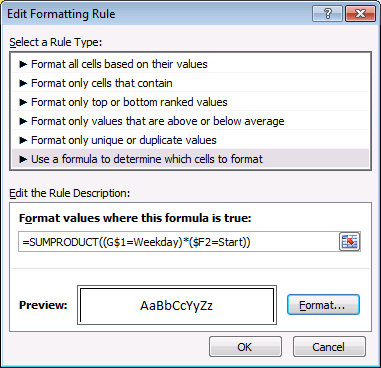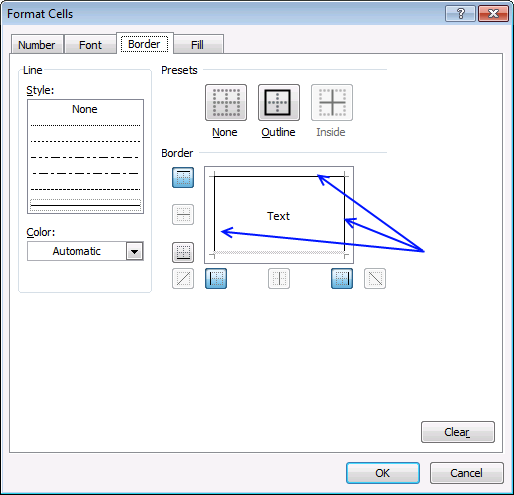Author: Oscar Cronquist Article last updated on March 22, 2019

This template makes it easy for you to create a weekly school schedule, simply enter the time ranges and the formula takes care of the rest. See the animated image above.

The time ranges are entered in an Excel defined Table that expands automatically when new values are added, no need for dynamic named ranges.

The template has hours divided into 10-minute intervals, follow the instructions below and you will with ease create another interval if you prefer to use that.

If you don't like the look of the ranges I am using you can easily change the conditional formatting color that highlights the ranges in the schedule.

There is a workbook for you to download at the very end of this article.

### How I created this dynamic template

The schedule contains formulas, conditional formatting, and an Excel defined Table, it updates instantly when you add/delete new records.

#### Weekdays

1. Select cell G1
2. Type Monday
3. Select cell H1
4. Type Tuesday

Repeat above steps for remaining cells and weekdays in cell range H1:M1.

#### 10-minute intervals

1. Select cell F2
2. Type 8:00
3. Select cell F3
4. Type 8:10
5. Select cell range F2:F3

If you want to use a 12-hour clock AM/PM then simply select all time values in column F and press CTRL + 1 to open a dialog box that allows you to change cell formatting.

Click "Time" and then pick a format that you prefer.

1. Select cell A1
2. Type Weekday
3. Select cell B1
4. Type Subject
5. Select cell C1
6. Type Start
7. Select cell D1
8. Type End

#### Create Excel defined Table

1. Select cell A1.
2. Press CTRL + T to create an Excel defined Table.

My table looks like this:

It contains some random data.

#### Enter array formulas

1. Select cell G2
2. Click in the formula bar3. Type:
=IFERROR(IF(SUMPRODUCT((Table1[Start]=\$F2)*(G\$1=Table1[Weekday]))=0, "", INDEX(Table1[Subject], SUMPRODUCT((Table1[Weekday]=G\$1)*(Table1[Start]=\$F2)*(MATCH(ROW(Table1[Weekday]), ROW(Table1[Weekday])))))), "")
4. Press and hold Ctrl + Shift
5. Press Enter

#### Copy array formula

1. Select cell G2
2. Copy cell (Ctrl +c)
3. Select cell range H2:M2
4. Paste (Ctrl + v)
5. Select cell range G2:M2
6. Copy (Ctrl + c)
7. Select cell range C3:M50
8. Paste (Ctrl + v)

#### Create conditional formatting

1. Select cell range G2:M50
2. Go to tab "Home"
3. Click "Conditional Formatting" button
4. Click "New Rule..."
5. Click "Use a formula to determine which cells to format"
6. Type =SUMPRODUCT((G\$1=Weekday)*(\$F2=>Start))
in "Format values where this formula is TRUE" field.7. Click "Format..." button
8. Click Border tab
9. Click to create three borders10. Click OK
11. Click OK

Repeat above steps with remaining conditional formatting rules:

The border and fill formatting are also shown in the picture above.

1 : =SUMPRODUCT((G\$1=Weekday)*(\$F2>=Start)*(\$F2<End))

2: =SUMPRODUCT((G\$1=Weekday)*(\$F2=End))

3: =SUMPRODUCT((G\$1=Weekday)*(\$F2=Start))

4: =SUMPRODUCT((G\$1=Weekday)*(\$F2>=Start)*(\$F2<End))